# Operational Amplifier

## The operational amplifier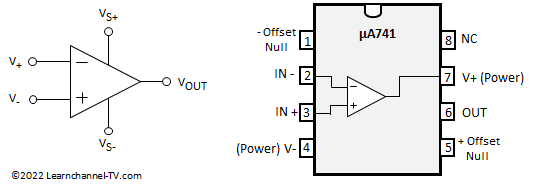Symbol Operational Amplifier, IC 741

The Operational Amplifier with appropriate circuitry is more than just an amplifier. It can transform signals and generate them itself, such as square-wave voltage or triangular signals. As an impedance converter it can be found in input circuits. More about this in this chapter.

### Operational Amplifier as Impedance Converter

In this circuit, the operational amplifier functions as an impedance converter due to its high input resistance and low output resistance. This allows a low impedance load to be connected to a signal source without putting too much load on the signal source.
Another name for this circuit is also voltage follower, because here the operational amplifier has an amplification factor of V = 1.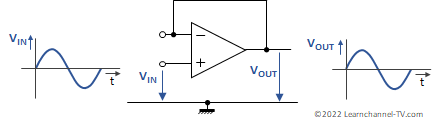Operational Amplifier as Impedance Converter

To explain this, one must know how the operational amplifier works: The operational amplifier strives to ensure that both of its inputs always have the same potential. At this point one also speaks of a "virtual zero point". This condition is only fulfilled here if the output voltage is equal to the input voltage.

### Inverting Operational Amplifier - Inverting Op Amp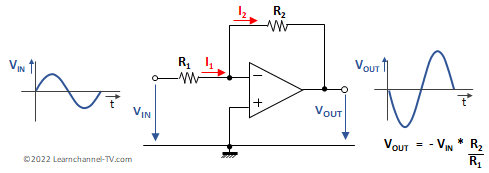Inverting Operational Amplifier - Inverting Op Amp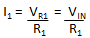This type of op-amp circuit produces an output which is 180° out of phase with its input.To explain this, let us again think of the virtual zero point at the inputs of the operational amplifier. If we now apply a voltage VIN to the input, a current I1 must inevitably flow: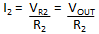Since the input resistance of the operational amplifier is very high, the input current of the OPV must also be negligibly low. This means that the current I1 must flow via R2.

Combining both equations, taking into account the direction of the current and you get: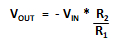### OP Amp as Non-Inverting Amplifier

Here the output signal is fed back to the inverting input by a voltage divider. The output signal is divided by the voltage divider, which results in an output signal being amplified. The resistance ratio determines the gain factor.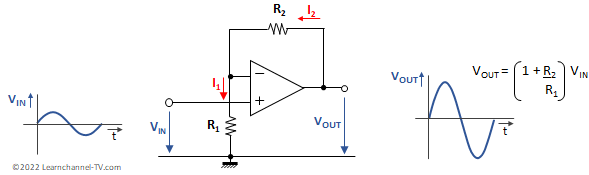Non-Inverting Operational Amplifier - Non-Inverting Op Amp

Since the input resistance of an operational amplifier is very high, the input currents are negligible. Thus: I1 = I2  (1)

Since the differential voltage at the inputs of the operational amplifier is zero, the following applies: VR1 = VIN      (2)

Apply the mesh rule and you get for the output voltage VOUT:

VOUT = VR1 + VR2   or    VOUT = VIN + VR2

With I1 = I2 and Ohm's law you get: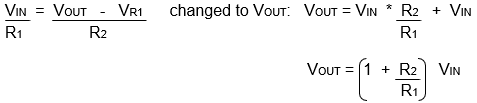### Operational Amplifier as Differential Amplifier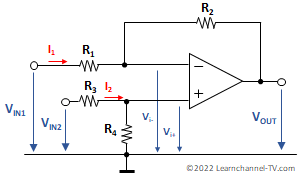Operational Amplifier as Differential Amplifier

Derivation of the equation for the output voltage considering that all 4 resistors R1 to R4 are of different values:

Case:  VIN1 = 0 and thus Vi- = 0:

Calculation of Vi+ via voltage divider: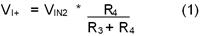For the partial output voltage VOUT+ you get: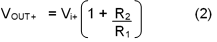Eq. (1) in Eq. (2) results in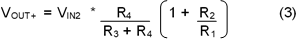Case: VIN2 = 0 and therefore Vi+ = 0: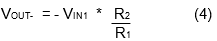Bringing together equations (3) and (4):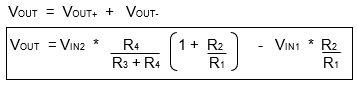If the input voltage in both inputs should be acquired in the same way:

The symmetry of the resistors must be right, i.e. the resistance ratios R1=R2 and R3=R4 must apply so that the operational amplifier, as a differential amplifier, acquires the same voltages at its inputs in the same way: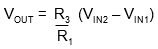### Operational amplifier as summer

An operational amplifier as a summer (Op-Amp as Summing Amplifier) can also perform additions. The summing amplifier is based on the principle of the inverting amplifier, with the difference that the input current is generated by two or more different input voltages. The input currents add up and are amplified as usual: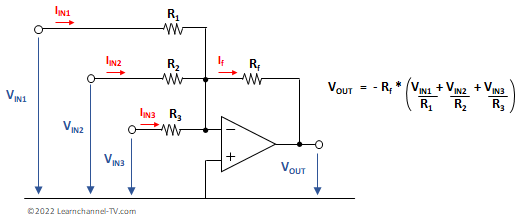Op-Amp as Summing Amplifier - Operational amplifier as summer

Since the inputs of the operational amplifier are at the virtual zero point and the differential voltage between the inputs is zero, the following applies:  Ve- = - VOUT

Since the input resistances of the operational amplifier are, as we know, very large, the input currents are negligible. Thus applies:  If = IIN1 + IIN2+ IIN3 + …

With Vf = Rf * If  and  Vf = - VOUT you get: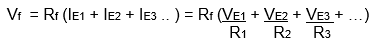Applications operational amplifier as a summer:

• Generation of mixed voltages
• Digital/analog converter

### Op-amp Integrator, Operational Amplifier Integrator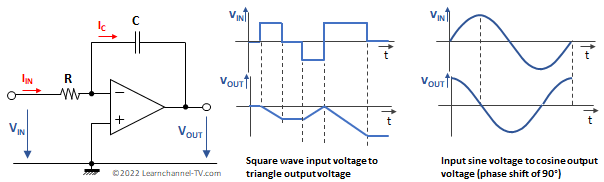Operational Amplifier as Integrator

An operational amplifier as an integrator has a capacitor as negative feedback. This results in a certain time and frequency behavior. If a constant input voltage VIN is applied, the capacitor charges via the resistor R1. The voltage Vc or VOUT thus increases steadily.

If the input voltage is sinusoidal, a phase shift of approx. 90 degrees can be seen at the output. This is due to the fact that the phase shift between current and voltage is 90 degrees for the capacitor.

Regarding frequency dependence: With increasing frequency, the capacitive reactance of the capacitor decreases and thus the voltage drop UC or UOUT. The integrator shows a low-pass behavior.

### Operational amplifier as Differentiator

The operational amplifier as a differentiator has a capacitor at its input. This input capacitor blocks DC voltage and low frequencies.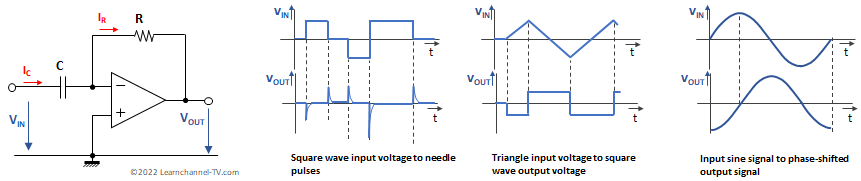Operational amplifier as Differentiator

The capacitor causes a phase shift between voltage and current of 90° (voltage lagging by 90°, i.e. -90°). Since the amplifier circuit additionally phase shifts the signal by 180°, the resulting phase shift at the output is +90°.

... soon coming more